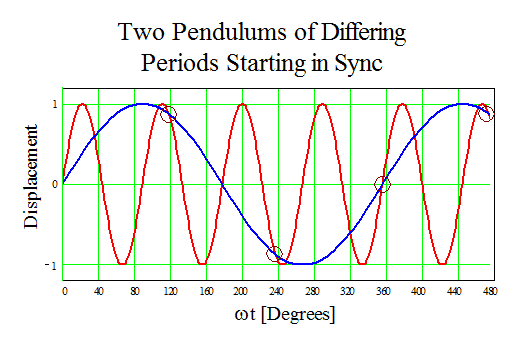# Pendulum oscillations problem

• Archived
Sir,
1) Two simple pendulums of length 1metre and 16 metre respectively are both given small displacements in the same direction at the same instant. They will again be in phase after the shorter pendulum has completed n oscillations. What is the value of n?

I solved a similar kind of problem in the following way:
2) Two pendula of lengths 121cm and 100cm start vibrating. At some instant they are in mean position in the same phase. After how many vibrations of the shorter pendulum, the two will be in the same phase in the mean position?
Let L(1) = 1.21 m
T(1) = 2(pie) (L(1)/g)^1/2 [2 pie root L one by g)
T(1) = 2.2 seconds

L(2) = 1.00 m
T(2) = 2(pie) (L(2)/g)^1/2 [Read as 2 pie root L two by g)
T(2) = 2 seconds
Since when they are in phase again the total time elapsed is the same,
T(1) * X(1) = T(2) * X(2)
X(2)/X(1) = T(1)/T(2)
= 2.2/2
= 1.1
= 11/10
So they will again be in phase when the shorter pendulum has completed 11 oscillations.
But this method doesn’t work with the first problem. Using the above method in the first problem, I get the answer as 4. But the answer given in my book is 4/3.

•Sourabh Yelluru

Amith2006 said:
Sir,
1) Two simple pendulums of length 1metre and 16 metre respectively are both given small displacements in the same direction at the same instant. They will again be in phase after the shorter pendulum has completed n oscillations. What is the value of n?

I solved a similar kind of problem in the following way:
2) Two pendula of lengths 121cm and 100cm start vibrating. At some instant they are in mean position in the same phase. After how many vibrations of the shorter pendulum, the two will be in the same phase in the mean position?
Let L(1) = 1.21 m
T(1) = 2(pie) (L(1)/g)^1/2 [2 pie root L one by g)
T(1) = 2.2 seconds

L(2) = 1.00 m
T(2) = 2(pie) (L(2)/g)^1/2 [Read as 2 pie root L two by g)
T(2) = 2 seconds
Since when they are in phase again the total time elapsed is the same,
T(1) * X(1) = T(2) * X(2)
X(2)/X(1) = T(1)/T(2)
= 2.2/2
= 1.1
= 11/10
So they will again be in phase when the shorter pendulum has completed 11 oscillations.
But this method doesn’t work with the first problem. Using the above method in the first problem, I get the answer as 4. But the answer given in my book is 4/3.

I suspect that you DO have the correct answer. n should be an integer. I think the book was giving the number of periods for both pendula...one goes 4 full cycles and the other goes 3.

-Dan

gneill
Mentor
A graphical demonstration is offered showing that the pendulums sync up before four full cycles of the faster pendulum, confirming the book's result of n = 4/3 is likely correct.

One pendulum has a period of 2 seconds and the other 8 seconds using the pendulum period formula:

##T = 2 \pi \sqrt{\frac{L}{g}}##

So one has a frequency four times the other. If we plot displacement as a function of ωt for both then we have two sinewaves:The circled points are places after time = 0 where the displacements are the same and the pendulums are moving in the same direction, i.e., they are momentarily in sync again. The first such occurrence is at ωt = 120°, and corresponds to 4/3 periods for the faster pendulum (red curve in the image).

A fairly simple algebraic solution is also possible which yields the solution exactly, but it is left as an exercise for the astute reader•DrClaude
epenguin
Homework Helper
Gold Member
Unnecessary detail in yr answer may be obscuring simple view for you.
The only thing different between the two pendulums is their length.
You don't need to work out the whole detail to get the periods ratio.Which would be the same on another planet with different g.